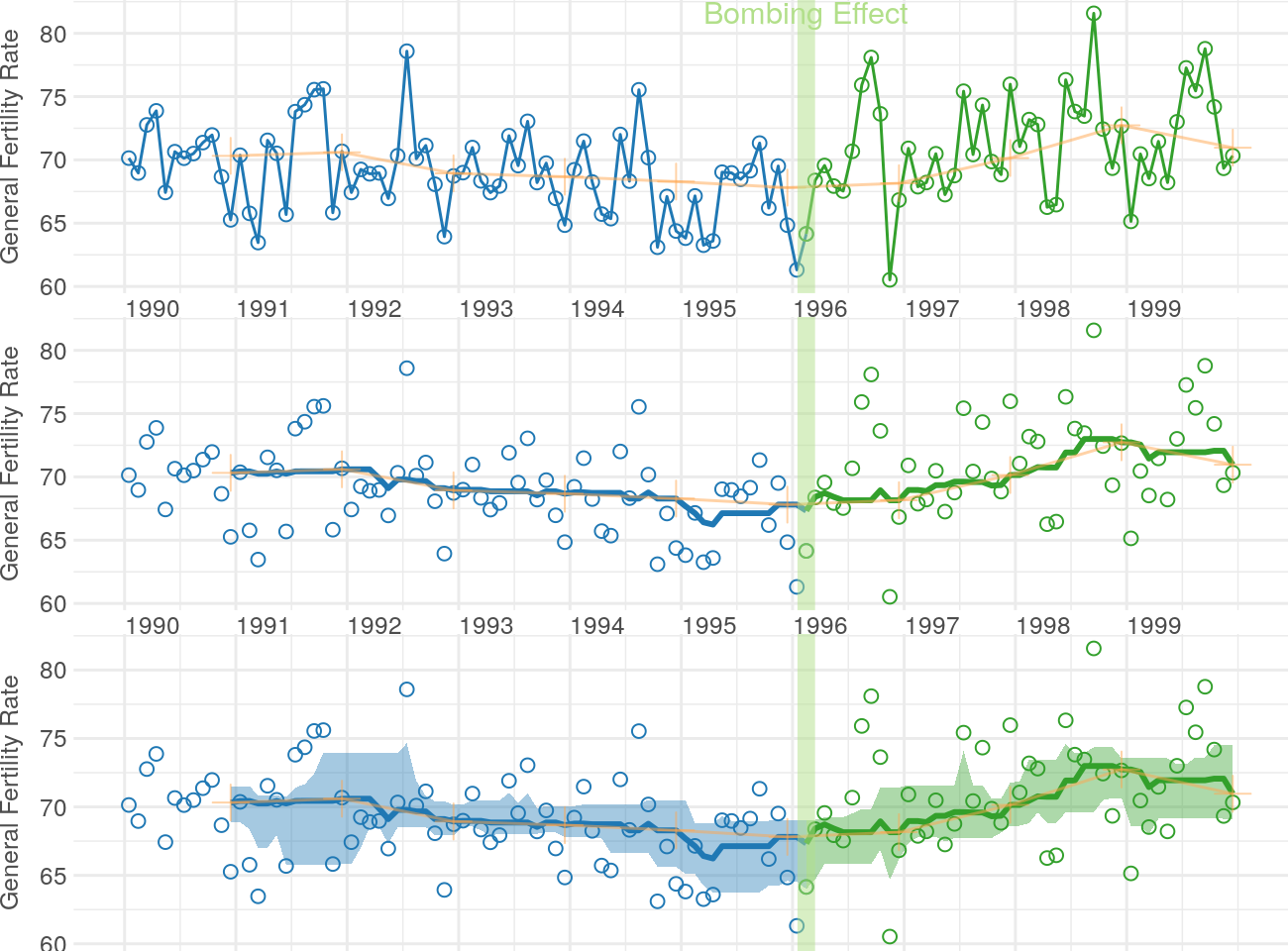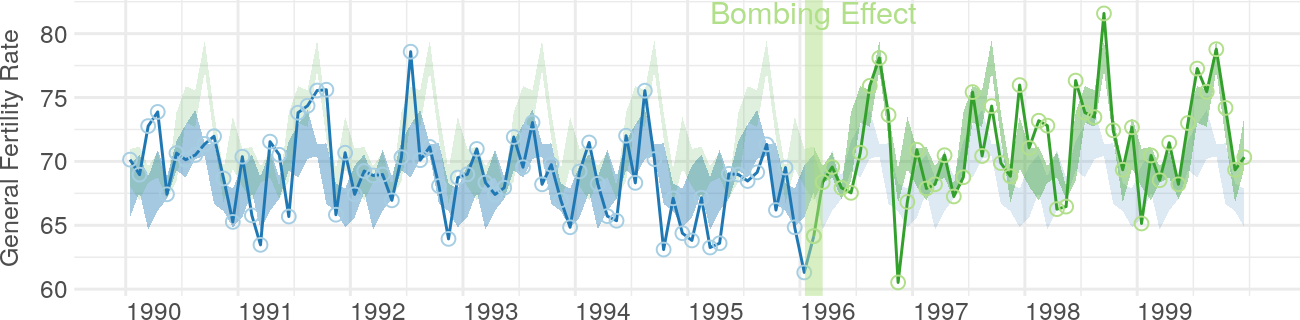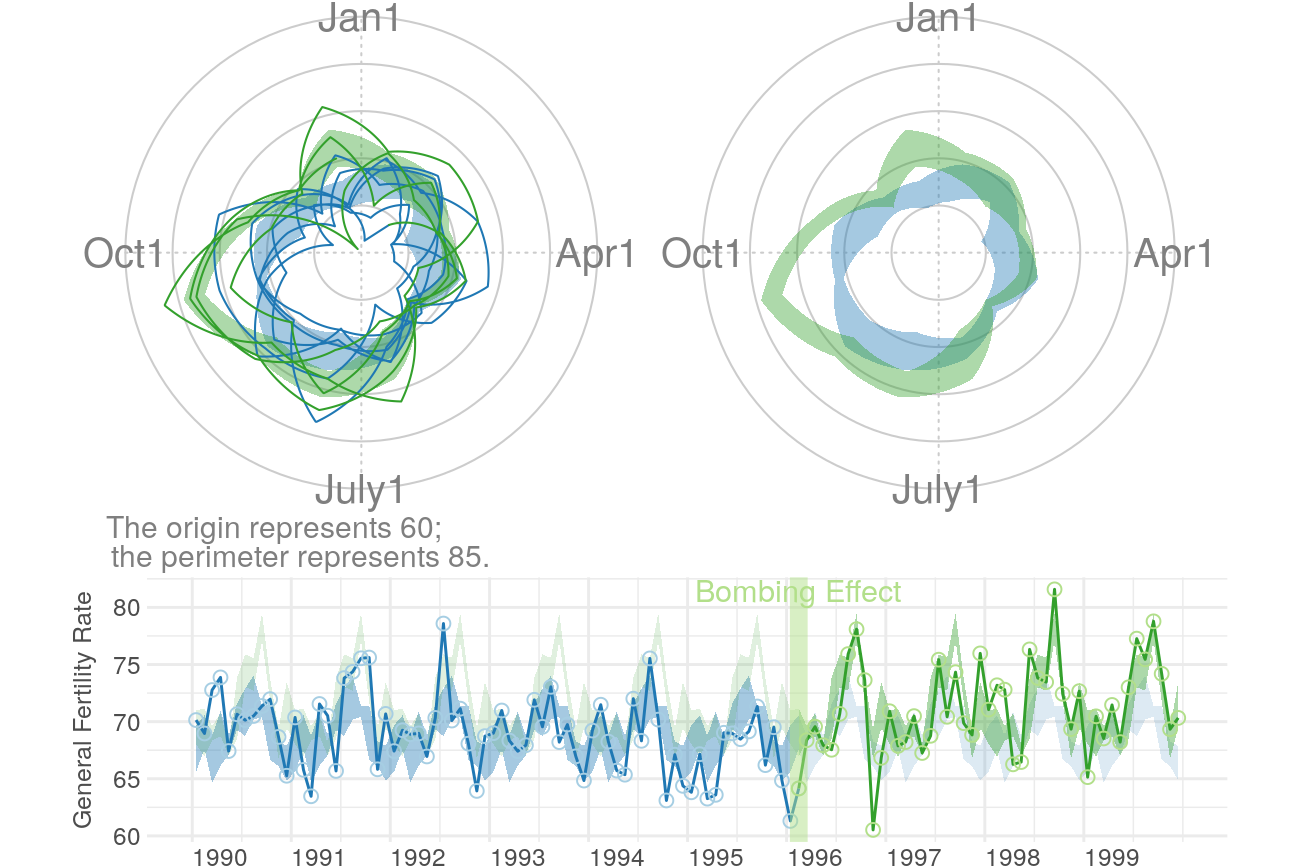# Assesing OKC Fertility with Intercensal Estimates

The MBR manuscript demonstrates WATS plots with data prepared for Rodgers, St. John, & Coleman (2005). In that paper and the MBR manuscript, the denominator of the GFR (General Fertility Rate) is the initial 1990 county population of females ages 15-44.

This vignette uses slightly different Census estimates. The intercensal population estimates (for females ages 15-44) are used for Jan 1990, Jan 1991, Jan 1992, …, Jan 2000. Linear interpolation is then used to complete the remaining 11 months of each year. These monthly estimates become the denominator of each county's monthly GFR.

``````changeMonth <- base::as.Date("1996-02-15") #as.Date("1995-04-19") + lubridate::weeks(39) = "1996-01-17"

vpLayout <- function(x, y) { grid::viewport(layout.pos.row=x, layout.pos.col=y) }

fullSpread <- function( scores ) {
return( base::range(scores) ) #A new function isn't necessary.  It's defined in order to be consistent.
}
hSpread <- function( scores ) {
return( stats::quantile(x=scores, probs=c(.25, .75)) )
}
seSpread <- function( scores ) {
return( base::mean(scores) + base::c(-1, 1) * stats::sd(scores) / base::sqrt(base::sum(!base::is.na(scores))) )
}
bootSpread <- function( scores, conf=.68 ) {
plugin <- function( d, i ) { base::mean(d[i]) }

distribution <- boot::boot(data=scores, plugin, R=99) #999 for the publication
ci <- boot::boot.ci(distribution, type = c("bca"), conf=conf)
return( ci\$bca[4:5] ) #The fourth & fifth elements correspond to the lower & upper bound.
}

darkTheme <- ggplot2::theme(
axis.title          = ggplot2::element_text(color="gray30", size=9),
axis.text.x         = ggplot2::element_text(color="gray30", hjust=0),
axis.text.y         = ggplot2::element_text(color="gray30"),
axis.ticks.length   = grid::unit(0, "cm"),
axis.ticks.margin   = grid::unit(.00001, "cm"),
#   panel.grid.minor.y  = element_line(color="gray95", size=.1),
#   panel.grid.major    = element_line(color="gray90", size=.1),
panel.margin        = grid::unit(c(0, 0, 0, 0), "cm"),
plot.margin         = grid::unit(c(0, 0, 0, 0), "cm")
)
``````
``````Warning: `axis.ticks.margin` is deprecated. Please set `margin` property of `axis.text` instead
``````
``````Warning: `panel.margin` is deprecated. Please use `panel.spacing` property instead
``````
``````lightTheme <- darkTheme + ggplot2::theme(
axis.title          = ggplot2::element_text(color="gray80", size=9),
axis.text.x         = ggplot2::element_text(color="gray80", hjust=0),
axis.text.y         = ggplot2::element_text(color="gray80"),
panel.grid.minor.y  = ggplot2::element_line(color="gray99", size=.1),
panel.grid.major    = ggplot2::element_line(color="gray95", size=.1)
)
dateSequence <- base::seq.Date(from=base::as.Date("1990-01-01"), to=base::as.Date("1999-01-01"), by="years")
xScale       <- ggplot2::scale_x_date(breaks=dateSequence, labels=scales::date_format("%Y"))
xScaleBlank  <- ggplot2::scale_x_date(breaks=dateSequence, labels=NULL) #This keeps things proportional down the three frames.
``````

## Section 1: Cartesian Rolling Plot

This section corresponds to the MBR manuscript, but with the modified dataset.

Smoothed monthly birth rates (General Fertility Rates; GFR's) for Oklahoma County, 1990-1999, plotted in a linear plot. The top plot shows the connected raw data with a February smoother; the middle plot shows smoothing with a 12-month moving average, blue/green line, superimposed on a February smoother, red tan line); the bottom plot shows the smoothers and confidence bands, which are H-spreads (ie, the distance between the .25 and .75 quantiles) defined using the distribution of GFR's for the given month and 11 previous months.

``````# dsLinear <- utils::read.csv("./Datasets/CountyMonthBirthRate2014Version.csv", stringsAsFactors=FALSE)
# dsLinear\$Date <- base::as.Date(dsLinear\$Date)
# dsLinear <- dsLinear[dsLinear\$CountyName=="oklahoma", ]

# Uncomment this line to use the version built into the package.  By default, it uses the
# CSV to promote reproducible research, since the CSV format is more open and accessible to more software.
dsLinearAll <- CountyMonthBirthRate2014Version
dsLinear <- dsLinearAll[dsLinearAll\$CountyName=="oklahoma", ]

dsLinear <- Wats::AugmentYearDataWithMonthResolution(dsLinear=dsLinear, dateName="Date")

topPanel <- Wats::CartesianRolling(
dsLinear = portfolioCartesian\$dsLinear,
xName = "Date",
yName = "BirthRate",
stageIDName = "StageID",
changePoints = changeMonth,
yTitle = "General Fertility Rate",
changePointLabels = "Bombing Effect",
drawRollingBand = FALSE,
drawRollingLine = FALSE
)

middlePanel <- CartesianRolling(
dsLinear = portfolioCartesian\$dsLinear,
xName = "Date",
yName = "BirthRate",
stageIDName = "StageID",
changePoints = changeMonth,
yTitle = "General Fertility Rate",
changePointLabels = "",
drawRollingBand = FALSE,
drawJaggedLine = FALSE
)

bottomPanel <- Wats::CartesianRolling(
dsLinear = portfolioCartesian\$dsLinear,
xName = "Date",
yName = "BirthRate",
stageIDName = "StageID",
changePoints = changeMonth,
yTitle = "General Fertility Rate",
changePointLabels = "",
drawJaggedLine = FALSE
)

topPanel <- topPanel + xScale + darkTheme
middlePanel <- middlePanel + xScale + darkTheme
bottomPanel <- bottomPanel + xScaleBlank + darkTheme

grid::grid.newpage()
grid::pushViewport(grid::viewport(layout=grid::grid.layout(3,1)))
print(topPanel, vp=vpLayout(1, 1))
print(middlePanel, vp=vpLayout(2, 1))
print(bottomPanel, vp=vpLayout(3, 1))
grid::popViewport()
``````## Section 2: Carteisan Periodic

This section corresponds to the MBR manuscript, but with the modified dataset.

Carteisan plot of the GFR time series data in Oklahoma County, with H-spread Bands superimposed.

``````cartesianPeriodic <- Wats::CartesianPeriodic(
portfolioCartesian\$dsLinear,
portfolioCartesian\$dsPeriodic,
xName = "Date",
yName = "BirthRate",
stageIDName = "StageID",
changePoints = changeMonth,
changePointLabels = "Bombing Effect",
yTitle = "General Fertility Rate",
drawPeriodicBand = TRUE #The only difference from the simple linear graph above
)
``````
``````Warning: Ignoring unknown aesthetics: y

Warning: Ignoring unknown aesthetics: y

Warning: Ignoring unknown aesthetics: y

Warning: Ignoring unknown aesthetics: y
``````
``````cartesianPeriodic <- cartesianPeriodic + xScale + darkTheme
print(cartesianPeriodic)
``````## Section 3: Polar Periodic

This section corresponds to the MBR manuscript, but with the modified dataset.

Wrap Around Time Series (WATS Plot) of the Oklahoma City GFR data, 1990-1999

``````portfolioPolar <- Wats::PolarizeCartesian(
dsLinear = portfolioCartesian\$dsLinear,
dsStageCycle = portfolioCartesian\$dsStageCycle,
yName = "BirthRate",
stageIDName = "StageID",
plottedPointCountPerCycle = 7200
)

grid::grid.newpage()
grid::pushViewport(grid::viewport(
layout=grid::grid.layout(
nrow = 2, ncol = 2, respect = TRUE,
widths = grid::unit(c(1,1), c("null", "null")),
heights = grid::unit(c(1,.5), c("null", "null"))
),
gp = grid::gpar(cex=1, fill=NA)
))

## Create top left panel
grid::pushViewport(grid::viewport(layout.pos.col=1, layout.pos.row=1))
topLeftPanel <- Wats::PolarPeriodic(
dsLinear = portfolioPolar\$dsObservedPolar,
dsStageCyclePolar = portfolioPolar\$dsStageCyclePolar,
stageIDName = "StageID",
cardinalLabels = c("Jan1", "Apr1", "July1", "Oct1")
)
grid::upViewport()

## Create top right panel
grid::pushViewport(grid::viewport(layout.pos.col=2, layout.pos.row=1))
topRighttPanel <- Wats::PolarPeriodic(
dsLinear = portfolioPolar\$dsObservedPolar,
dsStageCyclePolar = portfolioPolar\$dsStageCyclePolar,
stageIDName = "StageID",
drawObservedLine = FALSE,
cardinalLabels = c("Jan1", "Apr1", "July1", "Oct1"),
originLabel = NULL
)
grid::upViewport()

## Create bottom panel
grid::pushViewport(grid::viewport(layout.pos.col=1:2, layout.pos.row=2, gp=grid::gpar(cex=1)))
print(cartesianPeriodic, vp=vpLayout(x=1:2, y=2)) #Print across both columns of the bottom row.
upViewport()
``````## Section 4: Confirmatory Analysis of Interrupted Time Series

The remaining two sections depart from the MBR manuscript analyses. Its goal is to determine if the significant findings of Rodgers, St. John, & Coleman still appear with the modified Census estimates. As shown below, the the post-bombing fertility is still significantly higher than the pre-bombing fertility.

This section uses an approach advocated by McLeod, Yu, & Mahdi (2011), which is consistent other articles, including Rodgers et al. (2005). There are two trends that are de-seasonalized. The first is the 'classic' approach which uses the observed trend line (see `decompose()` on CRAN). The second is a smoothed version, where a loess is passed through the observed data; this smoothed line is then de-seasonalized (see `stl()` on CRAN). Both approaches lead to comparable conclusions. The post-bombing fertility is significantly higher than the pre-bombing fertility (ie, the `step` coefficient is significantly more positive).

``````dsLinear <- Wats::AugmentYearDataWithMonthResolution(dsLinear=dsLinear, dateName="Date")

tsData <- stats::ts(
data = dsLinear\$BirthRate,
start = as.integer(dsLinear[1, c("Year", "Month")]),
end = as.integer(dsLinear[nrow(dsLinear), c("Year", "Month")]),
frequency = 12L
)

#Create unsmoothed and smoothed version
seasonalClassic <- stats::decompose(tsData)
plot(seasonalClassic)
``````